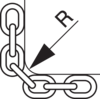Calculation of working load limit (WLL) for chain slings
When the exact information about weight, lifting angle and  position of the centre of gravity is known, you are able  to calculate the working load limit using the trigonometric method as follows:

2-leg sling
WLL (ton) = 2 x WLL for single leg sling x cos ß.

3-4-leg sling
WLL (ton) = 3 x WLL for single leg sling x cos ß.

Note: If the load is unform on all 4 legs in a sling the following formula can be used in exceptional cases:

WLL (ton) = 4 x WLL for single leg sling x cos ß.

ß = the legs angle against the vertical surface.

Sharp edges
Edge protectors should be used to prevent sharp edges from damaging the lifting equipment. As a general rule the radius (R) of the edge should be > 2x the chain diameter. When lifting with chain directly on lugs it is recommended that lug diameter greater than  3x the pitch of the chain should be used. If a lug diameter is less than this, the WLL must be reduced by 50%.

Edge R R > 2x chain Ø R > than chain Ø R = chain Ø
Celtspējas koeficients 1 0,7 0,5